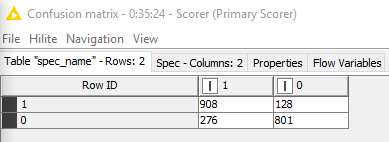# Confusion Matrix/Accuracy Statistics for learner model

Hi all,

While developing the classification model, We use the Learner and predictor node, The learner node, generates the model, based on supplied training set data and the predictor mode use the learner model and predict the test set data for accuracy/confusion matrix.

The RF and Treen ensemble learner node has port, where the confusion matrix or accuracy of learner model on training set data, can be explored. Now How can we get the same statitics for training set data in nodes like Decsion tree, NB, SVM, LibSVM.

Thanks

The "Scorer" node creates a confusion matrix and accuracy metrics.

P.

HI, thanks for the comment. Yes ""Scorer" node can get you confusion matric and accuracy metrics and this one can be obtained from predictor node by linking to Scorer node, but it will give the metrics for test set.

But i want to see the confusion matrics for trainig set data, so can u tell me where to link the "scorer" node to learner node specifically with Decision tree, NB and SVM learner node.

thanks

Scorer gives you the stats for ANY data set you feed it with. If you want optimize for a stat, you'll have to look into the optimizer nodes, which will involve Scorer in such a setting.

EDIT: I should have added what follows. To obtain the scores for the train set, you'll simply connect the Learner node to the Predictor node as usual and instead of feeding the test set to Predictor, you'll feed the train set this time. Then put a Scorer need next to it and there you go.

Thank you very much Geo...

I have noticed that depending on who you ask Confusion Matrix’ can be set up with the with Actual and Predicted Values on different Axis. I am pretty new to Data Science and it makes it pretty tough for me to understand. For KNIME Scorer Node are the Predicted Variables on the Left Side Column and the Actual Variables at the Top.Using the Scorer node configuration, if you use the true value in the first column and the prediction in the second column, then in the confusion matrix you will see the actuals in the rows and the predictions in the columns.

This is a little more explicit if you use the Scorer (Javascript) node instead:

Hope that helps.

2 Likes